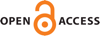Download this articleFor screen For printingRecent IssuesThe Journal About the Journal Editorial Board Editorial Interests Subscriptions Submission Guidelines Submission Page Policies for Authors Ethics Statement ISSN (electronic): 1472-2739 ISSN (print): 1472-2747 Author Index To Appear Other MSP Journals### Hans U Boden, Homayun Karimi and Adam S Sikora

Algebraic & Geometric Topology 23 (2023) 2271–2308##### Abstract

We apply Kauffman bracket skein algebras to develop a theory of skein adequate links in thickened surfaces. We show that any alternating link diagram on a surface is skein adequate. We apply our theory to establish the first and second Tait conjectures for adequate links in thickened surfaces. Our notion of skein adequacy is broader and more powerful than the corresponding notions of adequacy previously considered for link diagrams in surfaces.

For a link diagram $D$ on a surface $\mathrm{\Sigma }$ of minimal genus $g\left(\mathrm{\Sigma }\right)$, we show that

 $span\left({\left[D\right]}_{\mathrm{\Sigma }}\right)\le 4c\left(D\right)+4|D|-4g\left(\mathrm{\Sigma }\right),$

where ${\left[D\right]}_{\mathrm{\Sigma }}$ is its skein bracket, $|D|$ is the number of connected components of $D“$, and $c\left(D\right)$ is the number of crossings. This extends a classical result of Kauffman, Murasugi and Thistlethwaite. We further show that the above inequality is an equality if and only if $D$ is weakly alternating. This is a generalization of a well-known result for classical links due to Thistlethwaite. Thus, the skein bracket detects the crossing number for weakly alternating links. As an application, we show that the crossing number is additive under connected sum for adequate links in thickened surfaces.

##### Mathematical Subject Classification
Primary: 57K10, 57K12
Secondary: 57K14, 57K31
##### Publication